Courses

# Identify Class Language

## 15 Questions MCQ Test RRB JE for Computer Science Engineering | Identify Class Language

Description
This mock test of Identify Class Language for Computer Science Engineering (CSE) helps you for every Computer Science Engineering (CSE) entrance exam. This contains 15 Multiple Choice Questions for Computer Science Engineering (CSE) Identify Class Language (mcq) to study with solutions a complete question bank. The solved questions answers in this Identify Class Language quiz give you a good mix of easy questions and tough questions. Computer Science Engineering (CSE) students definitely take this Identify Class Language exercise for a better result in the exam. You can find other Identify Class Language extra questions, long questions & short questions for Computer Science Engineering (CSE) on EduRev as well by searching above.
QUESTION: 1

### The language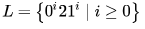over the alphabet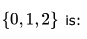Solution: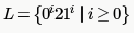has only one comparison that can be done using a DPDA. Hence, its DCFL.

Context free languages are a proper subset of Recursive Languages. ∴ it is recursive too.

QUESTION: 2

### Which of the following is true for the language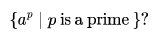Solution:

We have algorithms to generate prime numbers ⇒ we can generate sequence of P for the given language, hence
strings as defined by the language definition.

So by Church Turing Thesis we can say that there exists a Turing Machine which can accept the given language.

QUESTION: 3

### Consider the following languages.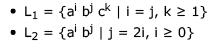Solution:

Both languages can be accepted by a DPDA :
L1 = start pushing element X into the stack on input 'a' ... start poping X on input 'b' ... move to final state when stack empty and input = 'c'

L2 = start pushing elements XX into the stack on input 'a' ... start poping X on input 'b' ... move to final state when stack
empty and input = 'epsilon'

so (A) and (D) are False

L1 ∪ L2 is a CFL ... we can build it by having L1, L2 and an extra state ... and an 'epsilon' transition to both L1 and L2 from
that extra state.

so (C) is false

L1 ∩ L2 = Phi because we have no string aibj where i=j and i=2j for i,j>=1
and clearly L1 is not a regular language

QUESTION: 4

Let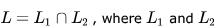are languages as defined below: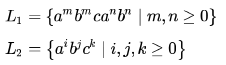Then L is

Solution: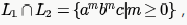which is context free but not regular.

QUESTION: 5

Consider the languages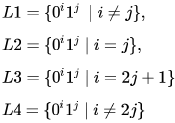Solution:

All are context free.
L1 -> Push 0 on stack and when 1 comes, start popping. If stack becomes empty and 1's are remaining start pushing 1. At
end of string accept if stack is non- empty.
L2 -> Do the same as for L1, but accept if stack is empty at end of string.
L3 -> Do, the same as for L2, but for each 0, push two 0's on stack and start the stack with a 0.
L4 -> Do the same as for L1, but for each 0, push two 0's on stack
All are in fact DCFL. Pushing two 0's on stack might sound non-trivial but we can do this by pushing one symbol and going
to a new state. Then on this new state on empty symbol, push one more symbol on stack and come back.

QUESTION: 6

Consider the languages L1,L2 and L3 as given below.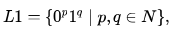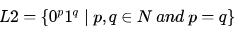and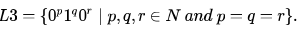Which of the following statements is NOT TRUE?

Solution: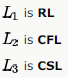Turning Machine is powerful Machine it can be used to accept all the languages (RL,CFL,CSL,RE)

QUESTION: 7

Consider the following languages.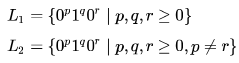Which one of the following statements is FALSE?

Solution:

L1 is regular and hence context-free also. Regular expression for L1 is 0*1*0*. So, (D) is the false choice.

L2 is context-free but not regular. We need a stack to count if the number of 0's before and after the 1 (1 may be absent also) are not same. So, L1 ∩ L2 is context-free as regular ∩ context-free is context-free. -> (B) is true.

Complement of L2 is recursive as context-free complement is always recursive (actually even context-sensitive).

QUESTION: 8

Consider the following languages over the alphabet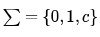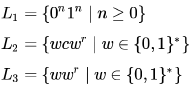Here, ωr is the reverse of the string . Which of these languages are deterministic Context-free languages?

Solution:

C.
L3 is CFL and not DCFL as in no way we can deterministically determine the MIDDLE point of the input string.

QUESTION: 9

Consider the context-free grammars over the alphabet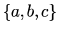given below.  S and T are non-terminals.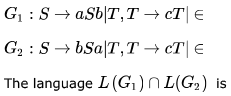Solution:

Since while intersection all strings produced by production aSb in G1 and bSa in G2 will be 0
so only common production will be
S-->T
T-->cT | epsilon
which is nothing but c* hence it is REGULAR and INFINITE

QUESTION: 10

Consider the following languages.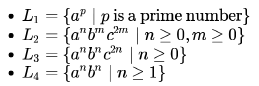Which of the following are CORRECT?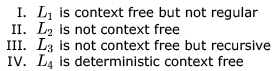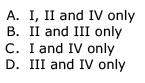Solution:

L1 is Csl,L2 is context free
L3 is not Context free and L4 is Dcfl

QUESTION: 11

Let L consist of all binary strings beginning with a 1 such that its value when converted to decimal is divisible by 5. Which of the following is true?

Solution:

l can be recognized by a dfa. we have a dfa to accept all such strings which when interpretated as decimal number are
divisible by n. where n can be anything the dfa of such can be made by a trick.
states are equal to possible remainders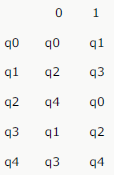if u can see the symmetry in it. write states and make fill like q0 q1 q2 q3 ...
now it is saying that it has to always start with 1 which the above dfa will not satisfy so make it a nfa by making a
transition from q0 on zero to a new dead state. now you have a nfa reduce it which will result in a deterministic dfa .

QUESTION: 12

Consider the following languages over the alphabet {0,1}.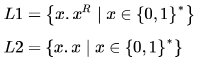Where xr is the reverse of string x; e.g. 011R =110 Which of the following is true?

Solution: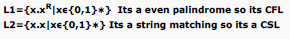QUESTION: 13

Let L be a given context-free language over the alphabet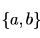. Construct L1 ,L2  as follows. Let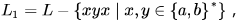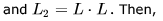Solution: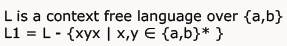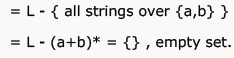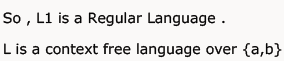L2 = L.L

Context free languages are closed under Concatenation.

So L2 is Context Free Language.

QUESTION: 14

Let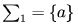be a one letter alphabet and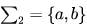be a two letter alphabet. A language over an alphabet is a set of finite length words comprising letters of the alphabet. Let L1 and L be the set of languages over  ∑1 and   ∑2    respectively. Which of the following is true about L1 and L:

Solution:

languages over alphabet set are uncountable so ans should be e

QUESTION: 15

Consider the following grammar  G with terminals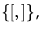start symbol S , and non-terminals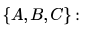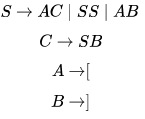A language L is called prefix-closed if for every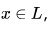every prefix of X is also in L . Which of the following is FALSE?

Solution:

The given grammar generates balanced parenthesis.

Lets take a smallest string : [ [ ] ] (say x )
Prefixes of x are : [ , [ [ ,[ [ ]

BUT they don't belong to the langauge generated by the given grammar.

SO, the answer will be Option D